﻿ 第二章 链表

## 2.1单向链表

typedef struct _node

{

int val;//值域，可以增加更多的值域，这里仅以int类型为代表

struct _node *next;//指针域，指向下一个结点，最后一个结点指向NULL

}Node, *PNode;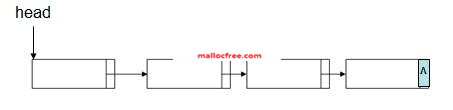### 2.1.1创建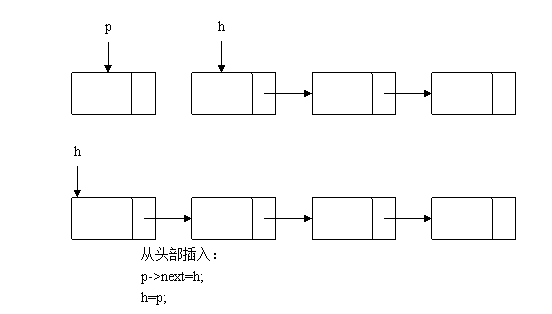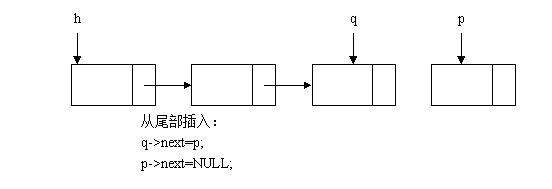//从头部插入创建链表算法

node *create_linklist_head()

{

node *h = NULL;

while(1)

{

node *p = (node*)malloc(sizeof(node));

if(p==NULL)

{

return h;

}

memset(p,0,sizeof(node));

printf("Please input a data\n");

scanf_s("%d",&p->value);

p->next = NULL;

if(h==NULL) //如果头结点指针为空，表明这是创建的第一个结点

{

h=p;

}

else

{

p->next = h;

h=p;

}

if(p->value==0)

{

break;

}

}

return h;

}

//从尾部插入链表算法

node *create_linklist_tail()

{

node *h=NULL;

while(1)

{

node *p = (node *)malloc(sizeof(node));

if(p==NULL)

{

return h;

}

memset(p,0,sizeof(node));

printf("Please input a data\n");

scanf_s("%d",&p->value);

p->next = NULL;

if(h==NULL) //如果头结点指针为空，表明这是创建的第一个结点

{

h=p;

}

else

{

node *q=h;//需要从头部遍历到尾部

while(q->next)

{

q=q->next;

}

q->next =p;//q为尾部，q->next=p，将新建结点设置为尾部

p->next = NULL;

}

if(p->value==0)//输入为零，则停止创建链表

{

break;

}

}

return h;

}

### 2.1.2插入

p->next=q->next;

q->next=p;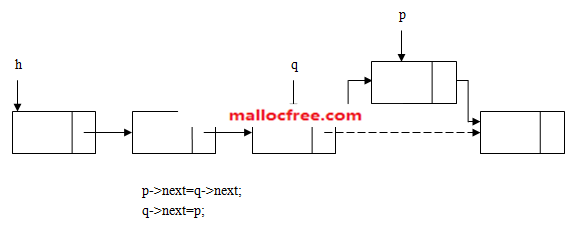### 2.1.3删除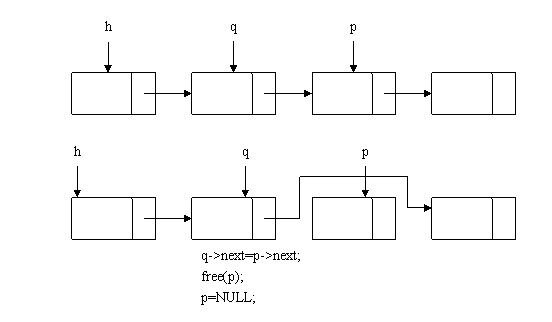void del_list_node(node **h,int value)

{

if(h==NULL)

{

return;

}

node *p = *h;

node *q=NULL;

while(p)

{

if(p->value==value)

{

if(p==*h)

{

*h=(*h)->next;

free(p);

return;

}

else

{

q->next=p->next;

free(p);

return;

}

}

q=p;

p = p->next;

}

}

### 2.1.4遍历

void traverse_list(node *h)

{

node *p = h;

while(p)

{

printf("%d ",p->value);

p=p->next;

}

printf("\n");

}

### 2.1.5销毁

void destroy_list(node *h)

{

node *p =h;

while(p)

{

node *q=p;//先用q保存这个待删除的结点

p=p->next;//p指向下一个结点

free(q);//现在可以删除q

}

}

## 2.2单向循环链表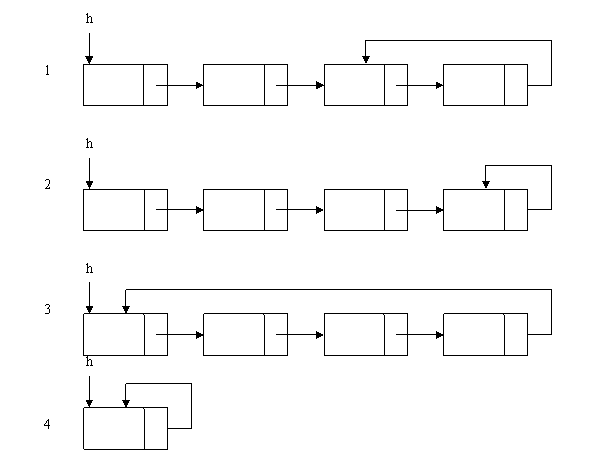//循环链表的创建：

node *create_list_loop()

{

node *h=NULL;

while(1)

{

node *p=(node *)malloc(sizeof(node));

if(p==NULL)

{

return h;

}

memset(p,0,sizeof(node));

printf("Please input a data\n");

scanf_s("%d",&p->value);

p->next=NULL;

if(h==NULL) //如果头结点指针为空，表明这是创建的第一个结点

{

h=p;

p->next=h;

}

else

{

node *q=h;

while(q->next!=h)//q->next如果等于h，那么q就是最后一个结点

{

q=q->next;

}

q->next = p;

p->next =h;//pnext指针指向h，构成一个循环

}

if(p->value==0)

{

break;

}

}

return h;

}

//循环链表的遍历方法，注意循环条件的判断：

void traverse_loop_list(node *h)

{

node *p=h;

do

{

printf("%d ",p->value);

p=p->next;

}while(p!=h);

printf("\n");

}

//循环链表的销毁方法

void destroy_loop_list(node *h)

{

node *p=h;

do

{

node *q=p;

p=p->next;

free(q);

} while (p!=h);

}

//步长法判断链表是否含有循环

//思路是：定义2个指针遍历该链表，1个指针跑一步，1个指针跑两步；

//那么如果两个指针相遇，则表示有循环，否则无循环。

bool is_a_loop_list(node *h)

{

node *p=h;

node *q=h->next;

while(p&&q&&q!=p&&q->next)

{

p=p->next;

q=q->next->next;

}

if(p==q)//循环退出，pq相等了，表示链表中存在循环

{

return true;

}

return false;

}

## 2.3双向链表

typedef struct _dnode

{

int data;

struct _dnode *pre;//前向指针，指向结点左边的结点

struct _dnode *next;//后继指针，指向结点右边的结点

}dnode, *pdnode;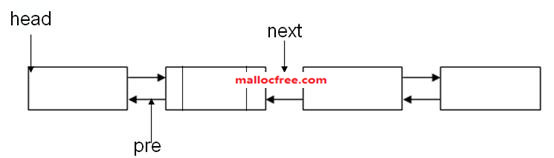### 2.3.1创建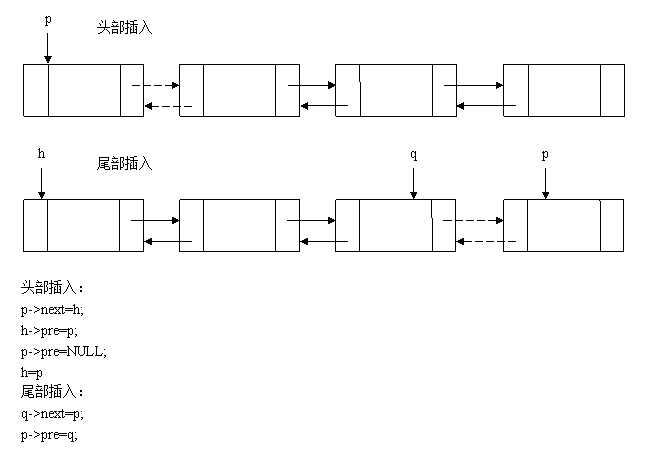//从头部插入方法创建双向链表：

dnode *create_dobulelist_head()

{

dnode *h = NULL;

while(1)

{

dnode *p=(dnode *)malloc(sizeof(dnode));

if(p==NULL)

{

return h;

}

memset(p,0,sizeof(p));

printf("Please input your data\n");

scanf_s("%d",&p->value);

p->next=p->pre=NULL;

if(h==NULL) //如果头结点指针为空，表明这是创建的第一个结点

{

h=p;

}

else

{

p->next = h;

h->pre=p;

h=p;

}

if(p->value==0)

{

break;

}

}

return h;

}

//从尾部插入创建双向链表：

dnode *create_dobulelist_tail()

{

dnode *h = NULL;

while(1)

{

dnode *p=(dnode *)malloc(sizeof(dnode));

if(p==NULL)

{

return h;

}

memset(p,0,sizeof(p));

printf("Please input your data\n");

scanf_s("%d",&p->value);

p->next=p->pre=NULL;

if(h==NULL)//如果头结点指针为空，表明这是创建的第一个结点

{

h=p;

}

else

{

dnode *q=h;

while(q->next)//需要先从头结点开始遍历到尾结点

{

q=q->next;

}

q->next = p;

p->pre=q;

p->next = NULL;

}

if(p->value==0)//创建循环退出

{

break;

}

}

return h;

}

### 2.3.2插入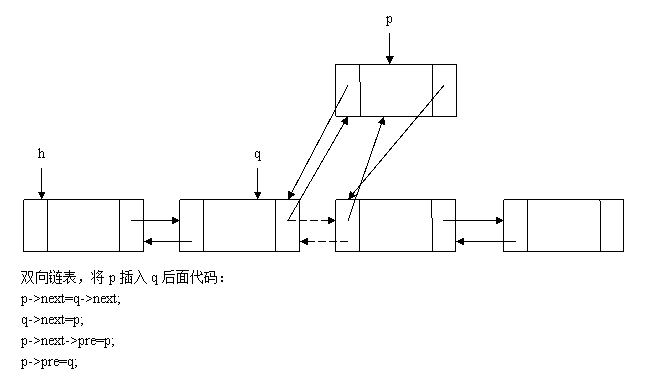### 2.3.3删除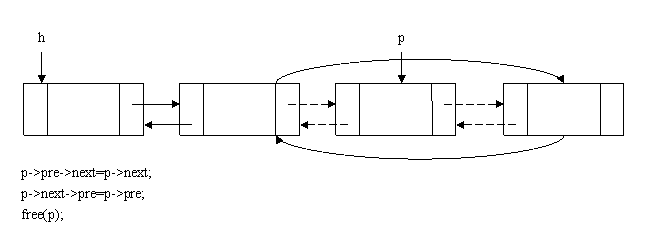### 2.3.4遍历

void traverse_dlist_next(dnode *h)

{

dnode *p=h;

while(p)

{

printf("%d ",p->value);

p=p->next;

}

printf("\n");

}

### 2.3.5销毁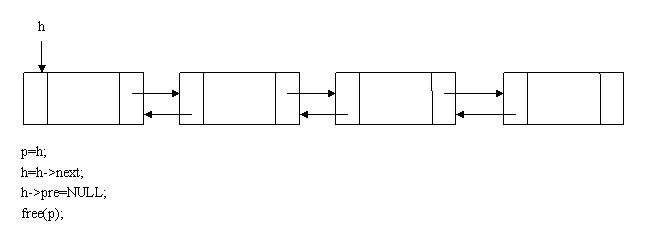void destroy_dlist(dnode *h)

{

dnode *p =h;

while(p)

{

dnode *q=p;

p=p->next;

free(q);

}

}

## 2.4双向循环链表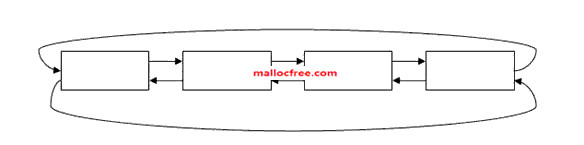p=h;

do {

p=p->next;

} while(p!=h)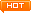看文字不过瘾？点击我，进入周哥教IT视频教学